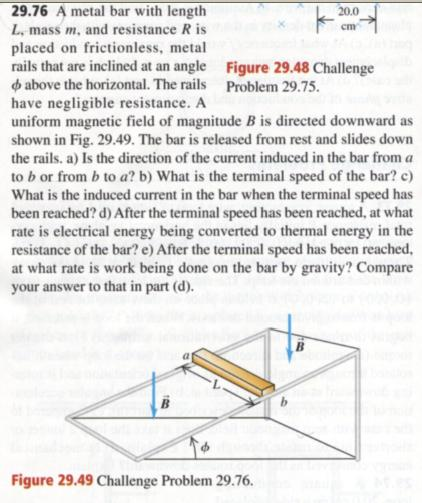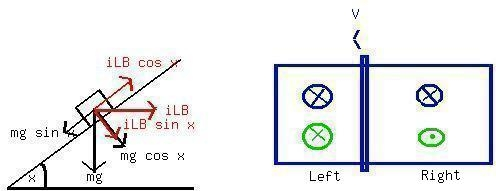Question# FSP02510401731.jpgFSZ

Other
ANSWERED2021-02-061. Refers to Lenz law :
The emf induced in an electriccircuit always acts in such a direction that the current it drivesaround a closed circuit produces a magnetic field which opposes thechange in magnetic flux.
a. Area in the right will experience more magnetic fluxentering its surface, that's why he produce magnetic fieldopposites to this change (exit the surface - dot marked)
b. Area in the left will experience less magnetic fluxentering its surface, that's why he produce magnetic fieldopposites to this change (enter the surface - crossmarked)
Right hand rule with your thumb indicate induced magneticfield and your remain finger indicate current direction we find that
current flows from a to b
b. according to Newton's law, terminal speed will be reachedwhen sum of force = 0, since there are two force acting on the baralong the path we have
$$\displaystyle{v}={\frac{{{m}{g}{R}{\sin{{x}}}}}{{{\left({B}{L}{\cos{{x}}}\right)}^{{2}}}}}$$
$$\displaystyle{I}={\frac{{{m}{g}{\sin{{x}}}}}{{{B}{L}{\cos{{x}}}}}}={\frac{{{m}{g}{\tan{{x}}}}}{{{B}{L}}}}$$
Power = $$\displaystyle{\left({\frac{{{m}{g}{\tan{{x}}}}}{{{B}{L}}}}\right)}^{{2}}\times{R}$$ Watt